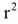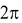# SPHERE AND HEMI-SPHERE

## Surface Area and Volume of Class 9

Sphere: Sphere is a three dimensional figure, which is made up of all points in the space, which lie at a constant distance called the radius, from a fixed point called the centre of sphere.

Hemi-sphere: If we exactly divide the sphere in two parts, then each part is known as hemi-sphere.

## SURFACE AREA OF SPHERE:

= 4πWhere r = radius of sphere.

### CURVED SURFACE AREA OF HEMI-SPHERE:

=Where r = radius of hemi-sphere.

### TOTAL SURFACE AREA OF HEMI-SPHERE

=+ π.

= 3πWhere r = radius of hemi-sphere.